Examples of the Complete-Data Inferences

For a given parameter of interest, it is not always possible to compute the estimate and associated covariance matrix directly from a SAS procedure. This section describes examples of parameters with their estimates and associated covariance matrices, which provide the input to the MIANALYZE procedure. Some are straightforward, and others require special techniques.

### Means

For a population mean vector, the usual estimate is the sample mean vector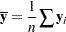A variance estimate for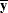is, whereis the sample covariance matrix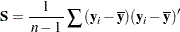These statistics can be computed from a procedure such as PROC CORR. This approach is illustrated in Example 57.2.

### Regression Coefficients

Many SAS procedures are available for regression analysis. Among them, PROC REG provides the most general analysis capabilities, and others like PROC LOGISTIC and PROC MIXED provide more specialized analyses.

Some regression procedures, such as REG and LOGISTIC, create an EST type data set that contains both the parameter estimates for the regression coefficients and their associated covariance matrix. You can read an EST type data set in the MIANALYZE procedure with the DATA= option. This approach is illustrated in Example 57.3.

Other procedures, such as GLM, MIXED, and GENMOD, do not generate EST type data sets for regression coefficients. For PROC MIXED and PROC GENMOD, you can use ODS OUTPUT statement to save parameter estimates in a data set and the associated covariance matrix in a separate data set. These data sets are then read in the MIANALYZE procedure with the PARMS= and COVB= options, respectively. This approach is illustrated in Example 57.4 for PROC MIXED and in Example 57.5 for PROC GENMOD.

PROC GLM does not display tables for covariance matrices. However, you can use the ODS OUTPUT statement to save parameter estimates and associated standard errors in a data set and the associated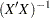matrix in a separate data set. These data sets are then read in the MIANALYZE procedure with the PARMS= and XPXI= options, respectively. This approach is illustrated in Example 57.6.

For univariate inference, only parameter estimates and associated standard errors are needed. You can use the ODS OUTPUT statement to save parameter estimates and associated standard errors in a data set. This data set is then read in the MIANALYZE procedure with the PARMS= option. This approach is illustrated in Example 57.4.

### Correlation Coefficients

For the population correlation coefficient, a point estimate is the sample correlation coefficient. However, for nonzero, the distribution ofis skewed.

The distribution ofcan be normalized through Fisher’stransformation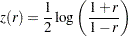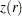is approximately normally distributed with mean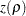and variance.

With a point estimateand an approximate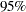confidence intervalfor, a point estimateand aconfidence intervalforcan be obtained by applying the inverse transformationto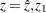, and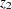.

This approach is illustrated in Example 57.10.

### Ratios of Variable Means

For the ratioof means for variables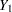and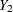, the point estimate is, the ratio of the sample means. The Taylor expansion and delta method can be applied to the functionto obtain the variance estimate (Schafer 1997, p. 196)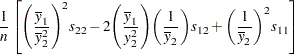whereand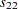are the sample variances ofand, respectively, and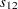is the sample covariance betweenand.

A ratio of sample means will be approximately unbiased and normally distributed if the coefficient of variation of the denominator (the standard error for the mean divided by the estimated mean) is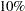or less (Cochran 1977, p. 166; Schafer 1997, p. 196).